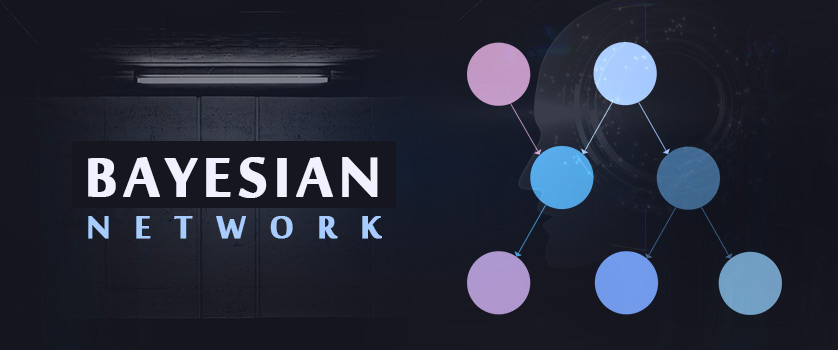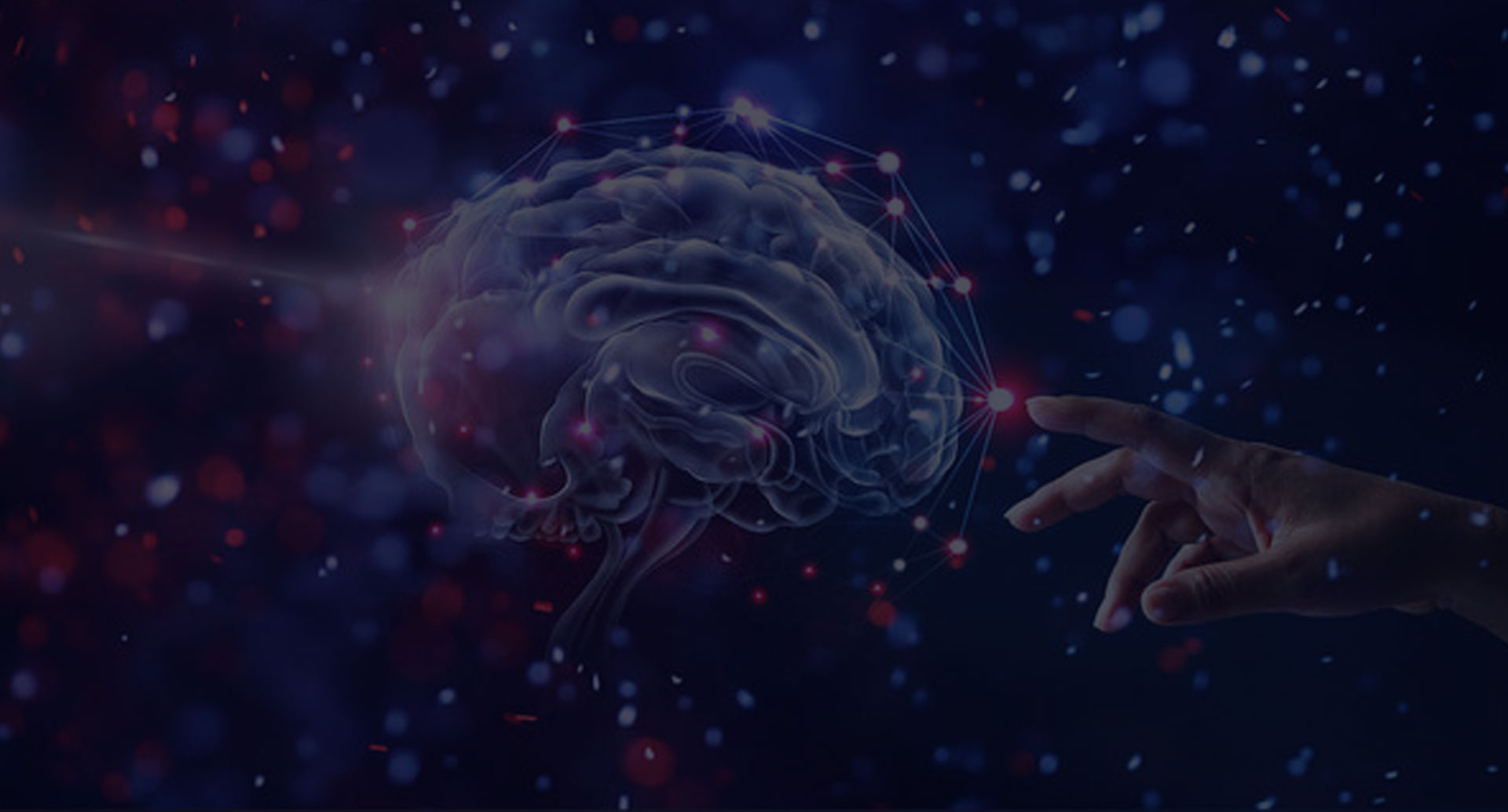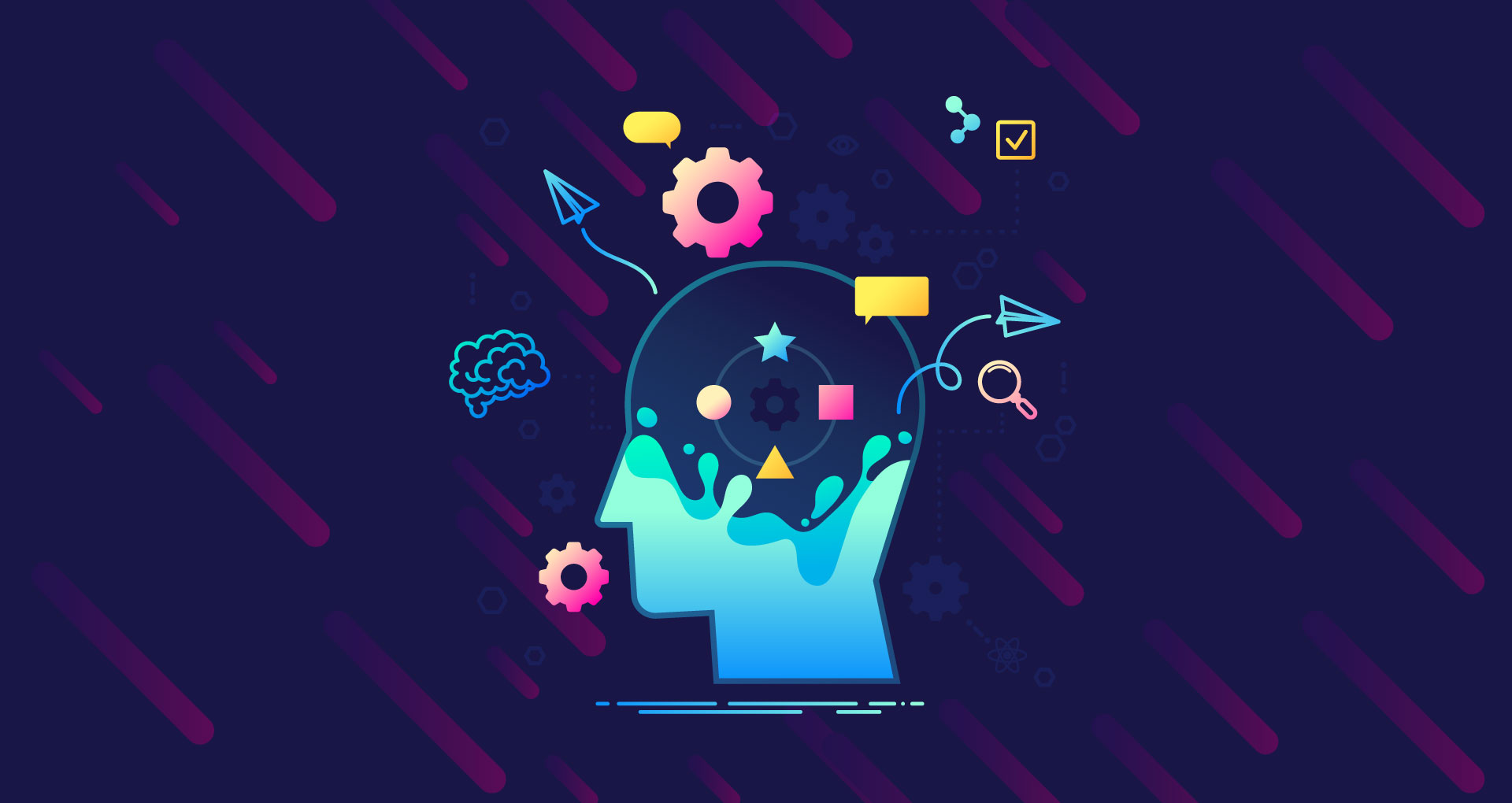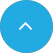# Bayesian NetworkApril 17, 2020

### Introduction:

In today’s tech-savvy world, Artificial Intelligence and its subfields machine learning, deep learning, etc. are enabling machines to perceive the external environment coherently. And to make this possible, these technologies are using multiple sources of information, which are efficiently merged to form a robust perception of the real-world. However, this stack of information and data can be uncertain and confusing, which becomes a hindrance in reaching the final result. Therefore, to deal with these uncertainties, different models and classifiers are used, one of which is the Bayesian Model or Bayesian Network.

A proven way for solving many challenging problems in several domains, Bayesian Network is effectively used for knowledge representation and reasoning under uncertainties. Since its importance is such in the field of Artificial Intelligence, let us further understand how Bayesian Network works as well as its application, architecture, and why Bayes Network is called Causal Network?

However, before indulging in a discussion on Bayesian Networks, we need to understand the basics of the Graphical Model.

### Graphical Model:

Defined in terms of a directed and undirected graph, Graphical Model is a type of probability distribution, which is also known as Probabilistic Graphical Model (PGM) or Structured Probabilistic Model. It is essentially a visualization of the chain rule. Here, nodes associated with random variables and joint probability distributions are used to define relations between them. It is further categorized into two types, based on whether the graph is directed or undirected:

• Bayesian Networks: Directed Graphical Model
• Markov Networks: Undirected Graphical Model

### What are Bayesian Networks?

Bayesian Network is a probabilistic graphical model that represents random variables and their conditional interdependencies through directed acyclic graphs (DAG). The term was first coined by Judea Pearl in 1985 to emphasize the subjective nature of the input data, the distinction

between the evidential and causal models of reasoning, and the dependency on Bayes’ conditioning for updating information.

Bayesian Network was named after Thomas Bayes (1702-1761), whose rule for updating probabilities considering new evidence became the foundation of the model. Currently, it is among the most widely used models that help reason with data uncertainties. Moreover, Bayesian Network is also known as Bayes Network, Belief Network, Decision Network, Bayes Model, and Probabilistic Directed Acyclic Graphical Model. Bayesian Networks are also termed as Causal Network when the requirement is that relationships between variables be causal.

### Bayesian Network Example:

The Bayesian Network can be used in a variety of scenarios, like to determine the probability of diseases an individual is suffering based on his/her symptoms. Other common examples where Bayesian Network analysis is used are:

• Bioinformatics.
• Speech recognition.
• Decision making.
• Profit maximization.
• Outcomes monitoring.
• Error detection.

### Features of Bayesian Networks:

The role of Bayesian Networks is not only limited to the above-defined functions. It also helps update beliefs about states of certain variables when a set of variables were observed and finds the most probable configurations of variables. Other important features that help define Bayesian networks are:

• It uses observed data to learn about the system’s structures and parameters.
• Represents relationships between a number of variables even in uncertain scenarios.
• Used in tasks like prediction, anomaly detection, reasoning, automated insight, etc.
• Uses the Bayesian Network inference for probability computation.
• Aims to model conditional independence by edges in a directed acyclic graph DAG.

### Components of Bayesian Networks:

A Bayesian Network consists of two important components, each of which enables it to perform the desired function. These two components are:

• Qualitative Component: It has Directed Acyclic Graph that explicates variables of interest, which is represented through nodes and the direct influences among them.
• Quantitative Component: Conditional Probability Distributions which helps quantify the dependencies between variables and their parents in the DAG using the expansion of variables in the joint probability function and conditional probability table.

It is with the help of these two components the Bayesian Network is able to specify the unique probability distributions over its variables as well as perform various inferences and learning.

The aspect that makes Bayesian Network important is that it has the capability to understand other techniques of Artificial Intelligence and Data Mining working without a joint probability distribution function. Additionally, it uses learning and inference to deduce properties about the probability distribution data. This involves three important tasks, which are:

• Inferring Unobserved Variables: Bayesian Networks can be used to answer probabilistic queries about variables and their relationships, as it is a complete model for them. It is a mechanism that automatically implements the Bayes’ theorem to find solutions to complex problems. This is accomplished with the help of various methods:
• Variable Elimination: It one-by-one removes the non-observed non-query variables by distributing the sum over the product.
• Clique Tree Propagation: Caches the computation to assess numerous variables at the same time and propagate new evidence quickly.
• Recursive Conditioning & AND/OR Search: Allows tradeoff of space-time and help estimate the efficiency of variable elimination when enough space is used. Apart from these the most commonly used approximate inference algorithms include importance sampling, generalized belief propagation, variational methods, mini-bucket elimination, stochastic MCMC simulation, & loopy belief propagation.
• Parameter Learning: The second inference task performed by the Bayesian network, parameter learning, is a process of using data to learn the distribution of a Bayesian network or Dynamic Bayesian Networks. Here, the Expectation-Maximization (EM) algorithm is used to perform maximum likelihood estimation, which enables the network to support
• Learning a subset of nodes or distributions
• Learning discrete & continuous distributions, etc.
• Structure Learning: This inference task performed by the Bayesian Network helps learn network structure and parameters of the local distributions from data. Moreover, it refers to learning the structure of the directed acyclic graph (DAG) from data. To accomplish these tasks, it uses two major approaches:
• Score-based approach: Specifies a statistical model and identifies high scoring structure by searching the space of networks. However, it requires heuristics as the space is super- exponential. It is essentially a search problem that consists of two parts:
• Score Metrics
• Search Algorithms
• Constraint-based approach: The second approach to structure learning, the constraint-based approach involves finding structures that best explain the determined dependencies by testing conditional dependence and independence in data structures. However, these are sensitive to failures in testing individual dependencies.

### Types of Bayesian Networks:

There are two basic types of Bayesian Networks for dynamic processes:

• Dynamic Bayesian Networks: A state-based Bayesian network, dynamic bayesian networks consist of a series of time slices that represent the state of all the variables at a certain time, t. This type of BN represents the temporal evaluation of a certain process as well as the state of each variable at discrete time intervals.
• Temporal Event Bayesian Networks: This is an event-based Bayesian network model that is an alternative to the dynamic bayesian networks for modeling dynamic processes. Here the time of occurrence of an event or change in variable state is represented through nodes. Furthermore, in cases where there are a few changes in the temporal range of events a more efficient representation is opted by the model such as Temporal Nodes Bayesian Networks.

### Bayesian Network Classifiers:

Classification is an integral part of decision making and pattern recognition and to ensure it is implemented accurately, researchers develop a range of classifiers that define them based on their attributes. Likewise, Bayesian Networks uses various classifiers to ensure the accuracy of the result. These are:

• Naive-Bayes Network: It is a network derived from Naive-Bayesian assumption and it does not consider the conditional interdependencies among the variables. It assumes each node is independent of one another.
• Tree Augmented Naive-Bayes network (TAN): A semi-naive Bayesian Learning method, tree augmented naive Bayes network takes into account all dependency relations between nodes in the Naive-Bayes network. Moreover, it has a complete tree structure for attribute classification.
• Bayesian Network Augmented Naive-Bayes network (BAN): This classifier takes a form of a network that encompasses local high or low-level nodes allowing a hierarchy specified between nodes.
• Bayesian Multi-network: Considered an extended version of TAN and BAN, it is useful when there is a need to produce a net depending on the different values of class nodes.
• General Bayesian network: General Bayesian Network of GBN is used to obtain meaningful class values without considering minor differences among the values.

### Steps to Develop Bayesian Network:

Another important aspect that we need to consider while defining the Bayesian network is how it is constructed. This involves five important steps that are:

• Model Objective: Define and specify the objectives for the model and the end-user as well as the system considerations and scales. This helps clarify system understanding and identify priorities
• Conceptual Model of the System: After defining the model objectives, the conceptual Bayesian Network is developed. This includes identifying important system variables and nodes as well as establishing links between them.
• Parameters the Model with Data: Once the conceptual model is developed, assign states and probabilities to each node and variable, which represents the potential values or conditions that a node can assume and helps provide the model necessary structure.
• Model Evaluation: To ensure the accuracy of the network, it is evaluated and tested using evaluation tools. However, this process can be time-consuming, especially in large networks.
• Scenario Analysis: Finally, Bayesian Networks can be used as decision support tools as they allow assessment of the relative changes in outcome probabilities and make predictions.

Now that we understand how the Bayesian network is developed, let us move on to understanding the Bayesian Neural Network.

### Application of Bayesian Networks:

The popularity of Bayesian Networks in the past few years has brought researchers and practitioners together to effectively and successfully implement it across industries. Today, Bayesian programming and application have become an integral part of deep learning software and applications. Therefore, to signify its impact, here are some real-world Bayesian Network applications are:

• Academics: The Learning Research and Development Center at the University of Pittsburgh developed an intelligent tutoring system for physics, Andes, using Bayesian Networks, which enabled them to assess and track student’s domain knowledge, over time. Moreover, Bayesian Network is helping the realm of academics to model the causal relation of student’s performance to classify them based on interventions and performance.
• Biology: The application of Bayesian Network is most evident in the field of biology, as it has helped researchers and practitioners to analyze gene expression data, infer gene networking, among other things. For example, Friedman et al developed a technique for learning causal relationships among genes with the help of Bayesian Networks.
• Medical & Healthcare: The value of Bayesian Network is great in medical and healthcare, as it solved several complex diagnostic problems encountered by the industry earlier.
• Business & Finance: The business and finance sector are benefiting through Bayesian Network, with organizations developing software for bayesian networks. Banks and organizations are using the Bayesian approach to classify bank loans, model and predict customer behavior in a variety of business settings, assess risks, etc. with limited or uncertain data.
• Computer Hardware & Software: Organizations like Intel, Microsoft, UT-Arlington and American Airlines, etc. have developed systems and applications with Bayesian Network to streamline and improve processes.

From handling stochastic events in a probabilistic framework to emphasizing only strong relations in the observed data, focusing on interactions with nodes directly affected by a small number of nodes there are a number of parameters and features that make Bayesian Network a viable and beneficial element of artificial intelligence. A few of these are:

• Transparently represents the causal components between system variables.
• Performs structural and parameter learning.
• It can be used as a visual decision support tool.
• Incorporates input data from different sources to overcome data limitations.
• Compactly represents large probability distributions.
• Uses inference algorithms to answer queries about distributions, without explicitly constructing them.

A technology still in its infancy, Bayesian Networks have not achieved complete perfection, irrespective of the advantages it offers. Additionally, it has certain disadvantages associated with it, which prevents its frequent adoption within organizations and industries, like:

• Performs continuous data representation.
• Lacks feedback loops.
• Deals with continuous variables only in a limited manner.
• It is difficult to collect and structure expert knowledge.
• Creating a simple but expressive probability distribution for local interaction is challenging.

### Bayesian Network Vs. Neural Networks

Though similar in look, there are certain qualities of both Bayesian and Neural Networks that set them apart from one another. Both are used as classifier algorithms and are directional graphs that take in a set of inputs for analysis and predict the output. However, the primary difference between the two is that the former has intrinsic meaning, whereas the latter does not.

Therefore to help you better understand their differences, here is a side by side comparison of the two:

### Bayesian Networks

• Its network structure offers valuable information about conditional dependence between the variables.
• Bayesian Networks represents independence and dependence relationships between variables.
• In the Bayesian Network graph each node represents a variable, and each directed edge represents a conditional relationship between variables.
• Bayesian Network is simpler than Artificial Neural Networks.

### Artificial Neural Network

• ANN’s network structure does not offer any valuable information for calculation.
• It does not have any direct interpretation, as the intermediate nodes of most neural networks are discovered features.
• Here, each node is a simulated "neuron" activated by the linear combination of the values of each output in the preceding network layer.
• Compared to Bayesian Network, ANN is more complicated.

### Conclusion:

Among the various classifiers used by AI and Machine Learning today like Gaussian Distributions, Logistic Representation, and more, Bayesian Network is a beneficial classifier that helps map relationships between actual numbers, events, and scenarios, in terms of probability. Moreover, it enables many intelligent machines and software to show and predict how certain scenarios influence the probability of an output. It is, in short, helping industries, especially medical & healthcare, to deal with uncertainties in data and reach new heights of diagnosis, prediction, and assessment.

### Popular PostAI Complete Guide.Artificial Neural Network.Machine Learning.